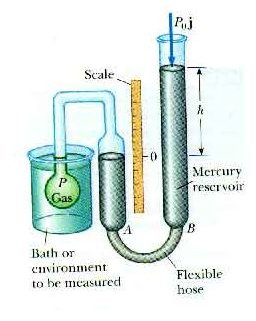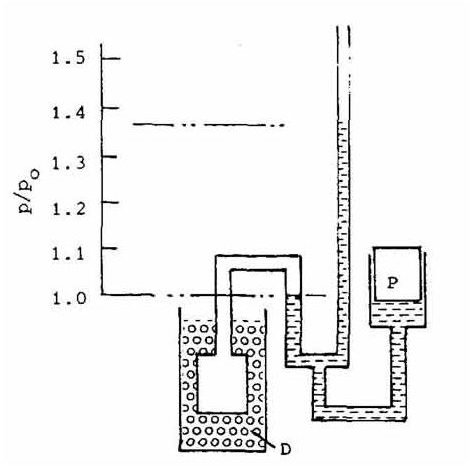# What is Constant Volume Gas Thermometer?

## Introduction

Thermometers are working examples of the zeroth law of thermodynamics. The significance of constant volume gas thermometers is that they are used to calibrate other thermometers.

## Construction

A constant volume gas thermometer is composed of a bulb filled with a fixed amount of a dilute gas that is attached to a mercury manometer. A manometer is a device used to measure pressure.The mercury manometer has a column partially filled with mercury that is connected to a flexible tube that has another partially filled column of mercury, called a reservoir, attached to the other end. The height of the mercury in the first column is set to a reference point or pressure P that it must stay at, while the mercury in the reservoir is allowed to move up and down in relation to a scale or ruler.

From the Law of Gay-Lussac, we know that when the temperature of an ideal gas increases, that there is a corresponding increase in pressure. Conversely, when the temperature decreases, so does the pressure. Constant volume gas thermometers operate on the same principle, with the restrictions that the gas is at low pressure and the temperature of it is well above that of liquefaction.

## Calculations

For a pressure P, the equation used to find the temperature T is

1) T = aP + b

where a and b are constants determined from two fixed points, such as ice at 0oC and steam at 100oC.

As mentioned previously, the constant volume gas thermometer uses values from the triple point of water to calibrate other thermometers. To recall, 273.16 K (Kelvin) is the temperature where water exists in an equilibrium state as a gas, liquid, and solid. For equation 1, where a = 273.16 K, b = 0, Ptp is the pressure of the gas at the triple point of water, and P is the pressure of the gas at the temperature to be measured, we have

2) T = 273.16 K (P/Ptp)

For low pressure and high temperatures, where real gases behave like ideal gases, equation 1 becomes:

3) T = 273.16 K lim P/Ptp as Ptp → 0

## Operation

The gas bulb is inserted into a bath or place we wish to find the temperature of, such as water. When the temperature increases or decreases, the volume also increases or decreases as well as the pressure, as we have seen in Charles’ Law and the aforementioned Gay-Lussac’s Law. The pressure of the mercury also changes such that it begins to move up or down and thus away from the reference point.To stop this movement, which will also stop the gas from expanding, the reservoir at the other end is physically lifted up or down, and the ensuing new pressure at this height is measured. The difference between the reference and reservoir heights gives the final pressure P, which is then used to calculate the temperature.

Figure 2 shows an example from Henry Sostmann’s Fundamentals of Thermometry text. A helium filled bulb is placed into a water and steam bath that is at 100oC. The mercury at the reference point is 1 Pa (Pascal), while that of the reservoir is at approximately 1.367 Pa. The difference between these two is used to help calculate the temperature of the bath.

## References

Physics for Scientists and Engineers by Douglas Giancoli

Physics For Scientists and Engineers by Raymond Serway

Image Credits

Constant Volume Gas Thermometer by Dr. Doug Davis Department of Physics Eastern Illinois University

Figure 2 from Henry Sostmann’s Fundamentals of Thermometry Part One (659K PDF File)